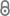# Optimizing Water Tanks in Water Distribution Systems by combining Network Reduction, Mathematical Optimization and Hydraulic Simulation

C. Hallmann, L. Suhl, OR Spectrum (2015) 1–19.263-Hallmann_-_Optimizing_water_tanks_in_water_distribution_systems.pdf 430.92 KB
Journal Article
Author
Hallmann, Corinna; Suhl, Leena
Abstract
In the last two decades, water consumption in Germany has been decreasing, which causes the water tanks and pipes in water distribution systems to work inefficiently. This paper proposes a method that supports the planning process for tanks in water distribution systems. The method uses a combination of network reduction, mathematical optimization and hydraulic simulation. The mathematical optimization model is a non-convex Mixed Integer Quadratically Constrained Program (MIQCP) that is solved by a piecewise linearization. As this may lead to many binary variables and therefore high computing times, the size of the water distribution system model is reduced before building the optimization model. After applying several network reduction techniques and using a piecewise approximation of the original model, there may be some hydraulic differences between the original network model and the reduced network model. To make sure that the solution obtained in the optimization process is feasible in the original water distribution system model, the solution is verified by a hydraulic simulation. If the solution is not feasible, the reduced model has to be modified and solved again until the hydraulic simulation verifies a solution as feasible. In this paper, each of these processes is described and the results indicate the usefulness of each of them.
Publishing Year
Journal Title
OR Spectrum
Page
1-19
LibreCat-ID

### Cite this

Hallmann C, Suhl L. Optimizing Water Tanks in Water Distribution Systems by combining Network Reduction, Mathematical Optimization and Hydraulic Simulation. OR Spectrum. 2015:1-19. doi:10.1007/s00291-015-0403-1
Hallmann, C., & Suhl, L. (2015). Optimizing Water Tanks in Water Distribution Systems by combining Network Reduction, Mathematical Optimization and Hydraulic Simulation. OR Spectrum, 1–19. https://doi.org/10.1007/s00291-015-0403-1
@article{Hallmann_Suhl_2015, title={Optimizing Water Tanks in Water Distribution Systems by combining Network Reduction, Mathematical Optimization and Hydraulic Simulation}, DOI={10.1007/s00291-015-0403-1}, journal={OR Spectrum}, publisher={Springer}, author={Hallmann, Corinna and Suhl, Leena}, year={2015}, pages={1–19} }
Hallmann, Corinna, and Leena Suhl. “Optimizing Water Tanks in Water Distribution Systems by Combining Network Reduction, Mathematical Optimization and Hydraulic Simulation.” OR Spectrum, 2015, 1–19. https://doi.org/10.1007/s00291-015-0403-1.
C. Hallmann and L. Suhl, “Optimizing Water Tanks in Water Distribution Systems by combining Network Reduction, Mathematical Optimization and Hydraulic Simulation,” OR Spectrum, pp. 1–19, 2015.
Hallmann, Corinna, and Leena Suhl. “Optimizing Water Tanks in Water Distribution Systems by Combining Network Reduction, Mathematical Optimization and Hydraulic Simulation.” OR Spectrum, Springer, 2015, pp. 1–19, doi:10.1007/s00291-015-0403-1.
Main File(s)
File Name
263-Hallmann_-_Optimizing_water_tanks_in_water_distribution_systems.pdf 430.92 KB
Access LevelClosed Access